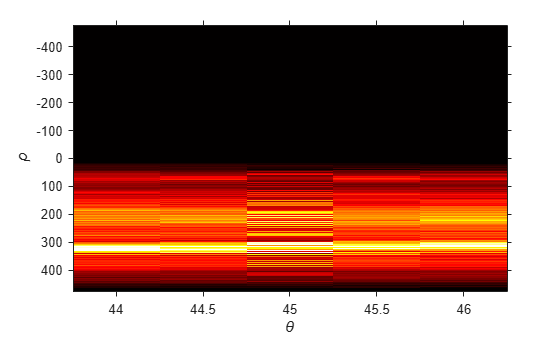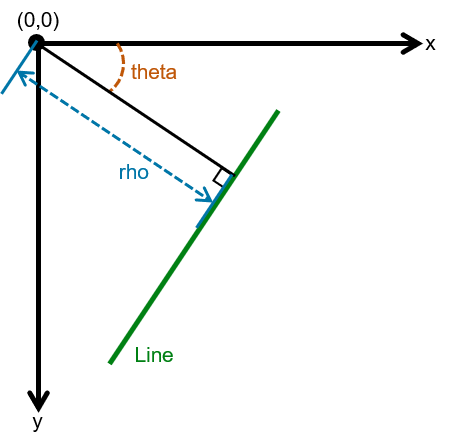Documentation

# hough

Hough transform

## Syntax

[H,theta,rho] = hough(BW)
[H,theta,rho] = hough(BW,Name,Value,...)

## Description

example

[H,theta,rho] = hough(BW) computes the Standard Hough Transform (SHT) of the binary image BW. The hough function is designed to detect lines. The function uses the parametric representation of a line: rho = x*cos(theta) + y*sin(theta). The function returns rho, the distance from the origin to the line along a vector perpendicular to the line, and theta, the angle in degrees between the x-axis and this vector. The function also returns the Standard Hough Transform, H, which is a parameter space matrix whose rows and columns correspond to rho and theta values respectively. For more information, see Algorithms.

example

[H,theta,rho] = hough(BW,Name,Value,...) computes the Standard Hough Transform (SHT) of the binary image BW, where named parameters affect the computation.

## Examples

collapse all

Read an image, and convert it to a grayscale image.

RGB = imread('gantrycrane.png'); I = rgb2gray(RGB);

Extract edges.

BW = edge(I,'canny');

Calculate Hough transform.

[H,T,R] = hough(BW,'RhoResolution',0.5,'Theta',-90:0.5:89);

Display the original image and the Hough matrix.

subplot(2,1,1); imshow(RGB); title('gantrycrane.png'); subplot(2,1,2); imshow(imadjust(rescale(H)),'XData',T,'YData',R,... 'InitialMagnification','fit'); title('Hough transform of gantrycrane.png'); xlabel('\theta'), ylabel('\rho'); axis on, axis normal, hold on; colormap(gca,hot);Read an image, and convert it to grayscale.

RGB = imread('gantrycrane.png'); I = rgb2gray(RGB);

Extract edges.

BW = edge(I,'canny');

Calculate the Hough transform over a limited range of angles.

[H,T,R] = hough(BW,'Theta',44:0.5:46);

Display the Hough transform.

figure imshow(imadjust(rescale(H)),'XData',T,'YData',R,... 'InitialMagnification','fit'); title('Limited Theta Range Hough Transform of Gantrycrane Image'); xlabel('\theta') ylabel('\rho'); axis on, axis normal; colormap(gca,hot)## Input Arguments

collapse all

Binary image, specified as a real, 2-D, nonsparse logical or numeric array.

Example: [H,T,R] = hough(BW);

Data Types: single | double | int8 | int16 | int32 | int64 | uint8 | uint16 | uint32 | uint64 | logical

### Name-Value Pair Arguments

Specify optional comma-separated pairs of Name,Value arguments. Name is the argument name and Value is the corresponding value. Name must appear inside quotes. You can specify several name and value pair arguments in any order as Name1,Value1,...,NameN,ValueN.

Example: [H,T,R] = hough(BW,'RhoResolution',0.5,'Theta',0.5);

Spacing of Hough transform bins along the rho axis, specified as the comma-separated pair consisting of 'RhoResolution' and a real, numeric scalar between 0 and norm(size(BW)), exclusive.

Data Types: double

Theta value for the corresponding column of the output matrix H, specified as the comma-separated pair consisting of 'Theta' and a real, numeric vector within the range [-90, 90).

Data Types: double

## Output Arguments

collapse all

Hough transform matrix, returned as a numeric array, nrho-by-ntheta in size. The rows and columns correspond to rho and theta values. For more information, see Algorithms.

Angle in degrees between the x-axis and the rho vector, returned as a numeric array of class double. For more information, see Algorithms.

Distance from the origin to the line along a vector perpendicular to the line, returned as a numeric array of class double. For more information, see Algorithms.

## Algorithms

The Standard Hough Transform (SHT) uses the parametric representation of a line:

rho = x*cos(theta) + y*sin(theta)

The variable rho is the distance from the origin to the line along a vector perpendicular to the line. theta is the angle of the perpendicular projection from the origin to the line measured in degrees clockwise from the positive x-axis. The range of theta is $-90°\le \theta <90°$. The angle of the line itself is $\theta +90°$, also measured clockwise with respect to the positive x-axis.The SHT is a parameter space matrix whose rows and columns correspond to rho and theta values respectively. The elements in the SHT represent accumulator cells. Initially, the value in each cell is zero. Then, for every non-background point in the image, rho is calculated for every theta. rho is rounded off to the nearest allowed row in SHT. That accumulator cell is incremented. At the end of this procedure, a value of Q in SHT(r,c) means that Q points in the xy-plane lie on the line specified by theta(c) and rho(r). Peak values in the SHT represent potential lines in the input image.

The Hough transform matrix, H, is nrho-by-ntheta where:

nrho = 2*(ceil(D/RhoResolution)) + 1, and
D = sqrt((numRowsInBW - 1)^2 + (numColsInBW - 1)^2).
rho values range from -diagonal to diagonal, where
diagonal = RhoResolution*ceil(D/RhoResolution).

ntheta = length(theta)

Download now